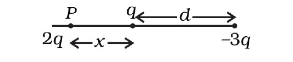# Two charges q and –3q are placed fixed

Question:

Two charges q and –3q are placed fixed on x-axis separated by distance‘d’. Where a third charge 2 should be placed such that it will not experience any force?

Solution:

The situation given in question is shown in the figure given below:At $P$ : on $2 q$, Force due to $q$ is to the left and that due to $-3 q$ is to the right.

$\Rightarrow \frac{2 q^{2}}{4 \pi \varepsilon_{o} x^{2}}=\frac{6 q^{2}}{4 \pi \varepsilon_{o}(d+x)^{2}}$

$\Rightarrow(d+x)^{2}=3 x^{2}$

$\Rightarrow(d+x)=\pm \sqrt{3} x$

$\Rightarrow x=\frac{d}{2} \pm \frac{\sqrt{3} d}{2}$

Here, ve sign would be between $q$ and $-3 q$ and hence it is not possible.

$\Rightarrow x=\frac{d}{2}+\frac{\sqrt{3} d}{2}=\frac{d}{2}(1+\sqrt{3})$ to the left of $q$